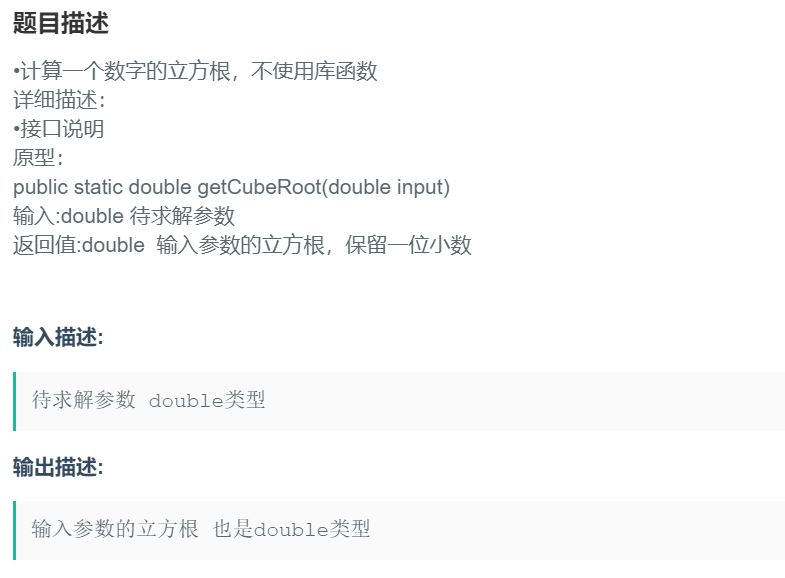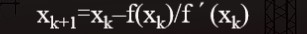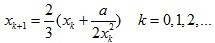## 求解一个数的立方根（想不懂都难）

【前面这一大段废话是两周前写的，现在才来干正事，我真是个废物】## 思路二：二分法

``````public static double getCubeRoot(double input)
{

if(input==0||input==1||input==-1)
return input;
else
{

double min = 0;
double max = input;
double mid = 0;
// 注意，这里的精度要提高一点，否则某些测试用例无法通过
while ((max - min) > 0.001)
{

mid = (max + min) / 2;
if (mid * mid * mid > input)
max = mid;
else if (mid * mid * mid < input)
min = mid;
else
return mid;
}
return max;//这种情况是：在满足精确度的条件下无精确值，选择max作为近似值来返回。
//同样也可以选择min作为近似值来返回
}
}
``````

mid=(min+max)/2;

## 思路三：牛顿迭代法``````public static void main(String[] args) {

double x,y,x1,x2;
Scanner in = new Scanner(System.in);
x = in.nextDouble();
System.out.println("请手动输入一个初始近似值");
x1 = in.nextDouble();
in.close();
x2= (x/x1/x1+2*x1)/3;    //牛顿迭代公式的第一次使用
y = fun(x2,x);
System.out.printf("%.1f",y);
}
public static double fun(double x2,double x)
{

if(Math.abs(x2*x2*x2-x)<0.0000001) //递归的结束条件
return x2;
else
return fun((x/x2/x2+2*x2)/3,x);//牛顿迭代公式的递归使用
}
``````

OK，终于把这个在草稿箱里躺了两周的文章写完了。睡觉咯，晚安。